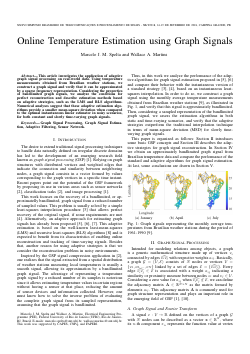Online Temperature Estimation using Graph Signals

Abstract
This article investigates the application of adaptive graph signal processing on real-world data. Using temperature measurements obtained from Brazilian weather stations, we construct a graph signal and verify that it can be approximated by a sparse frequency representation. Considering the properties of bandlimited graph signals, we analyze the conditions for perfect reconstruction and describe estimation methods based on adaptive strategies, such as the LMS and RLS algorithms. Numerical analyses suggest that these adaptive estimation algo- rithms provide a smaller mean-square deviation when compared to the optimal instantaneous linear estimator in noisy scenarios, for both constant and slowly time-varying graph signals.